# RS Aggarwal Solutions for Class 9 Chapter 2: Polynomials Exercise 2B

## RS Aggarwal Solutions for Class 9 Maths Exercise 2B PDF

In order to boost up the problem-solving capacity of the students to appear for the board exams, RS Aggarwal Solutions for class 9 can be used as a revision material. The solutions are prepared by subject experts based on the revised CBSE syllabus for Class 9. By using the PDF as a reference the students can solve the problems in less time with better understanding of the concepts and methods used in solving it. The main aim is to improve the problem-solving abilities of the students in order to appear for the board exams with high confidence. RS Aggarwal Solutions for Class 9 Maths Chapter 2 Polynomials Exercise 2B are provided here.

## RS Aggarwal Solutions for Class 9 Chapter 2: Polynomials Exercise 2B Download PDF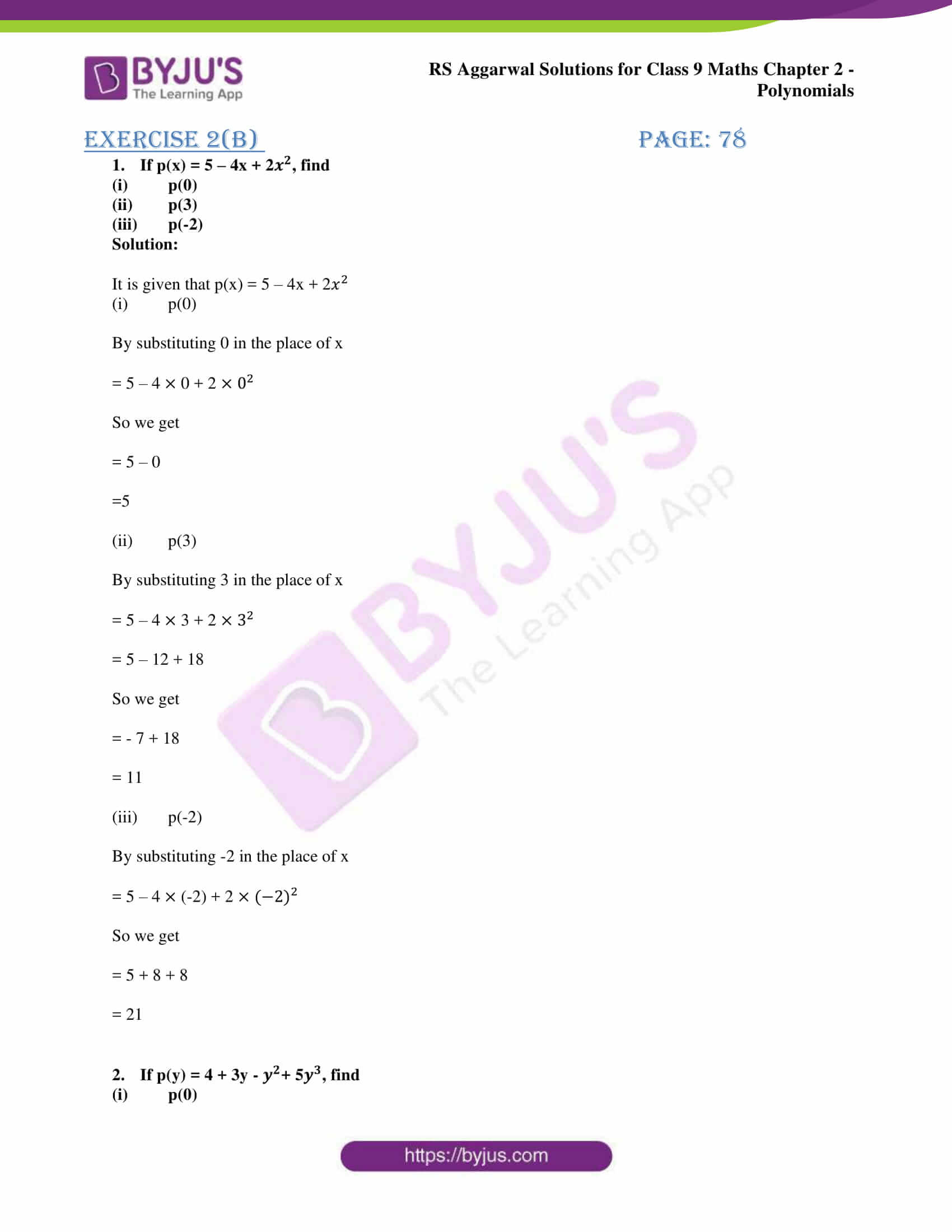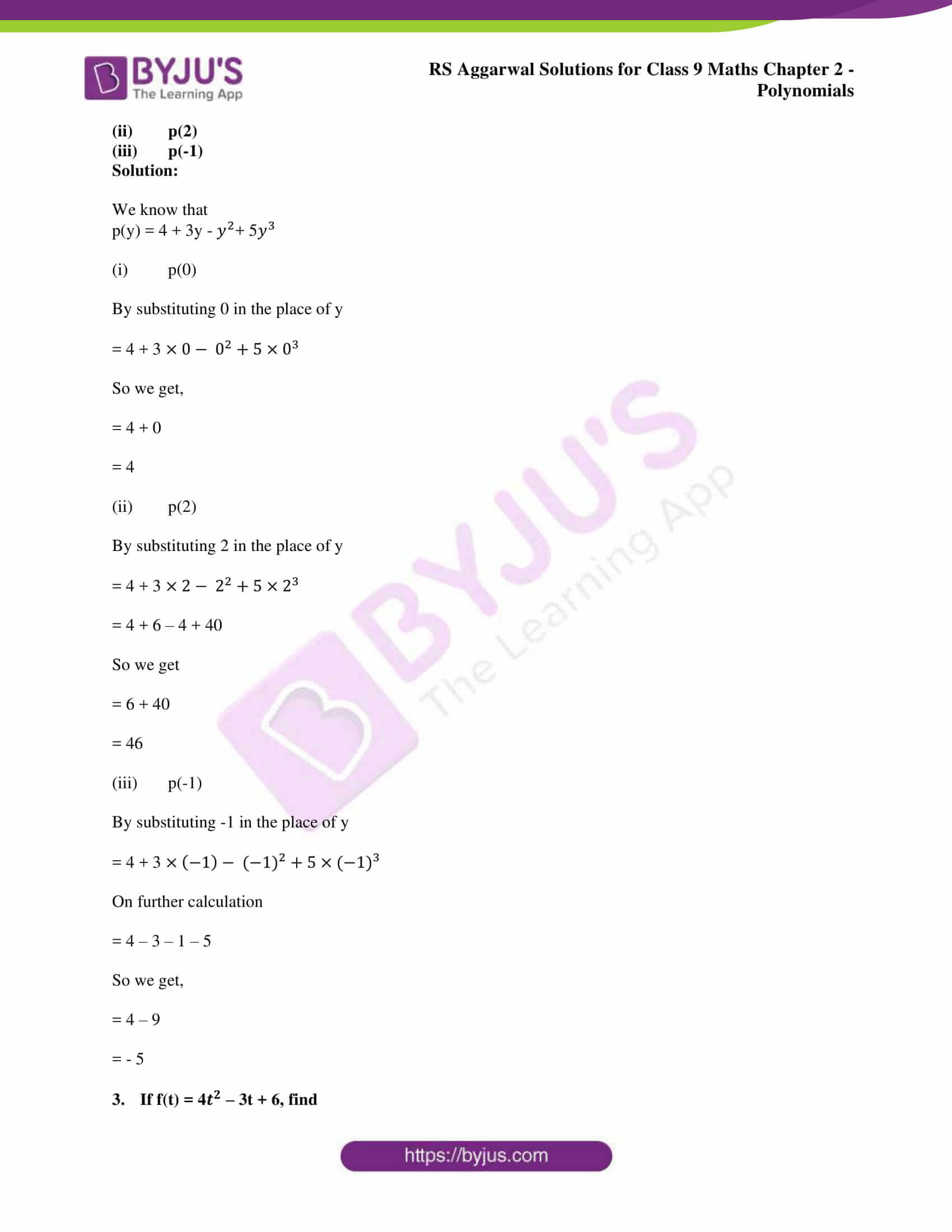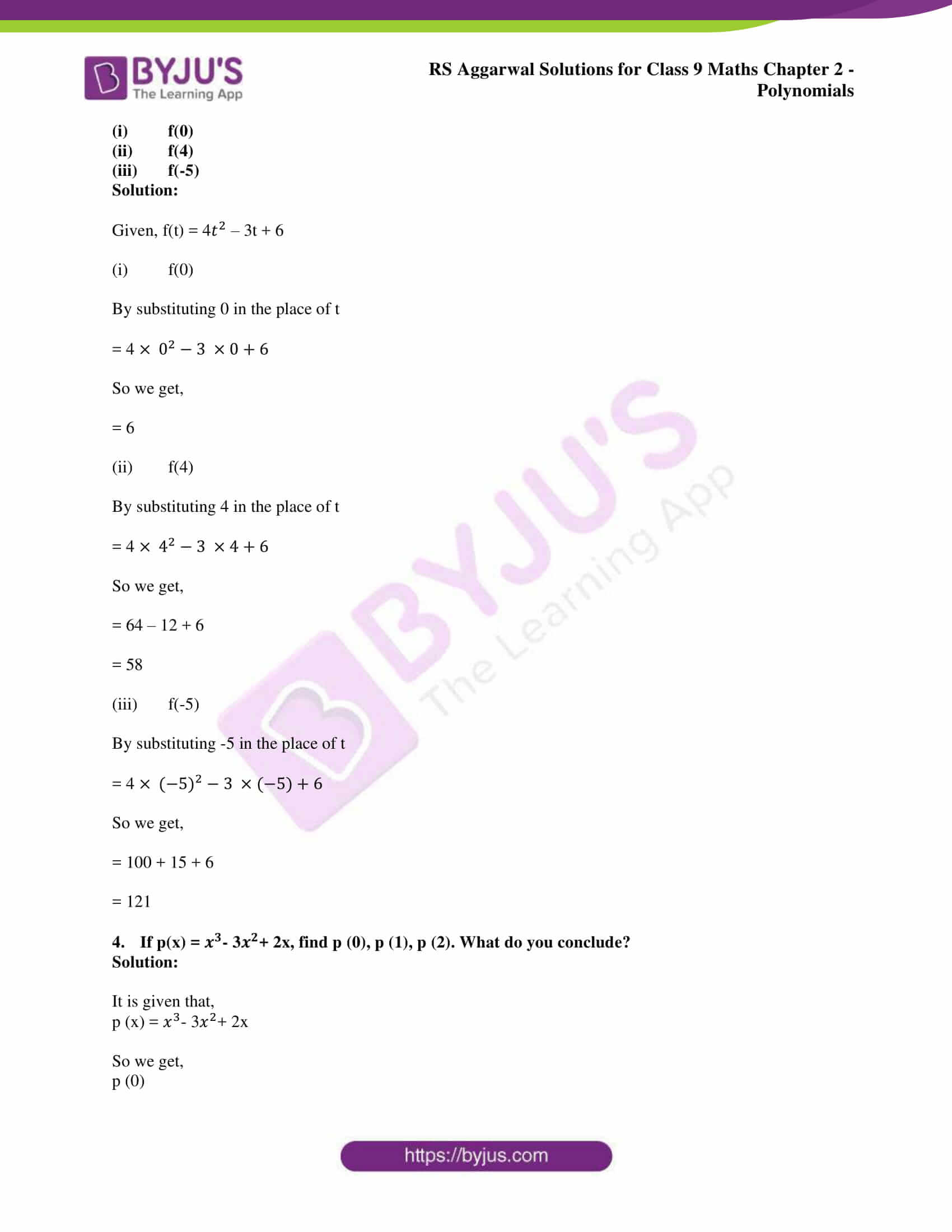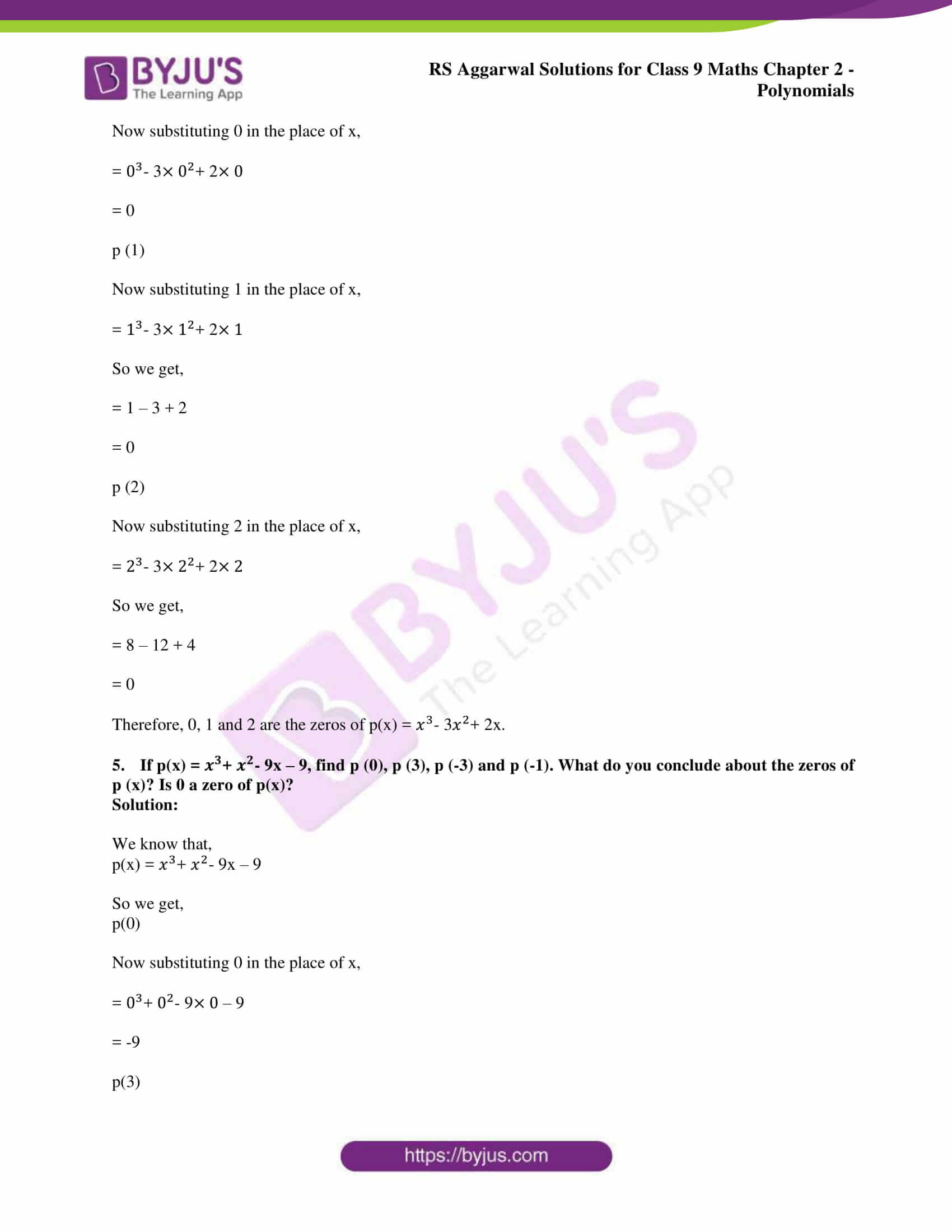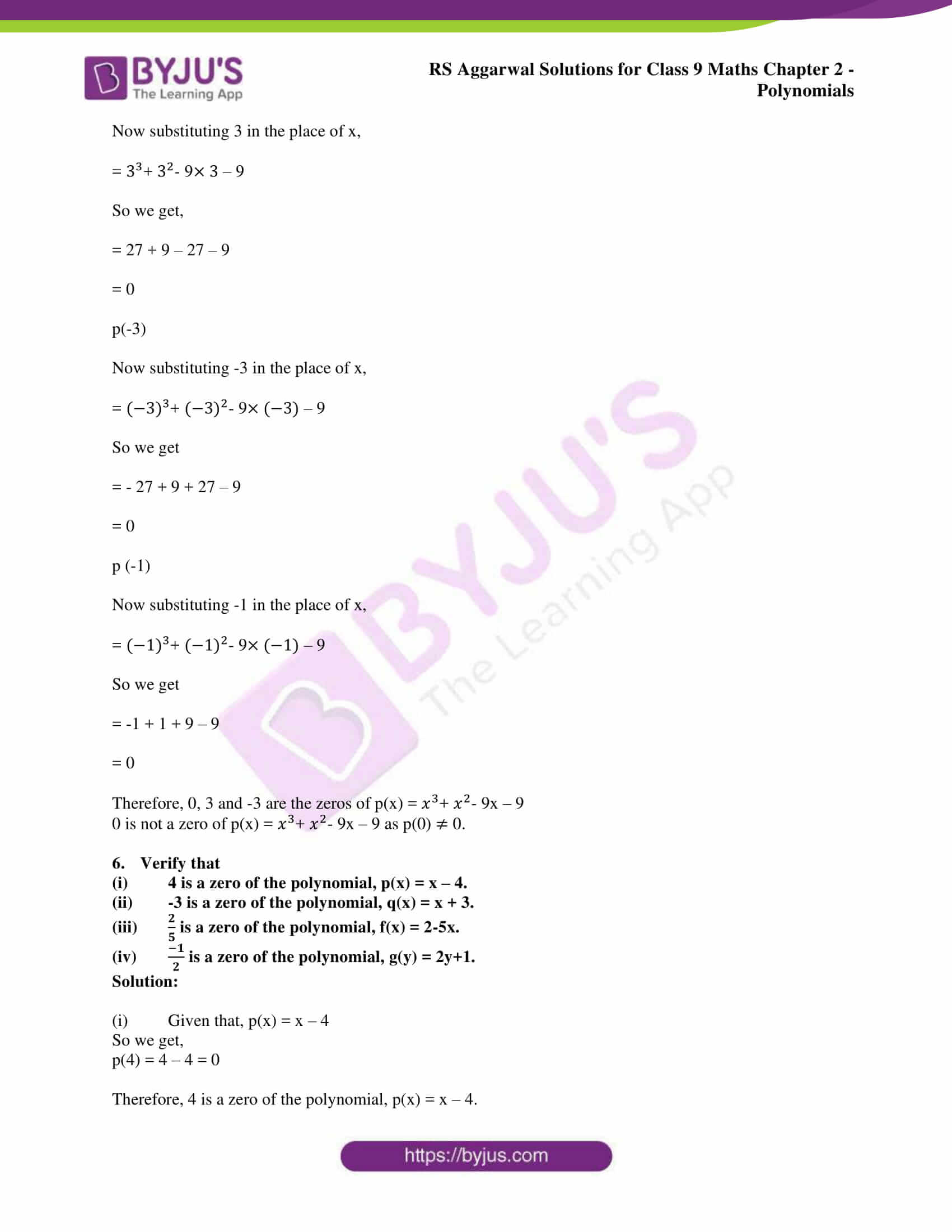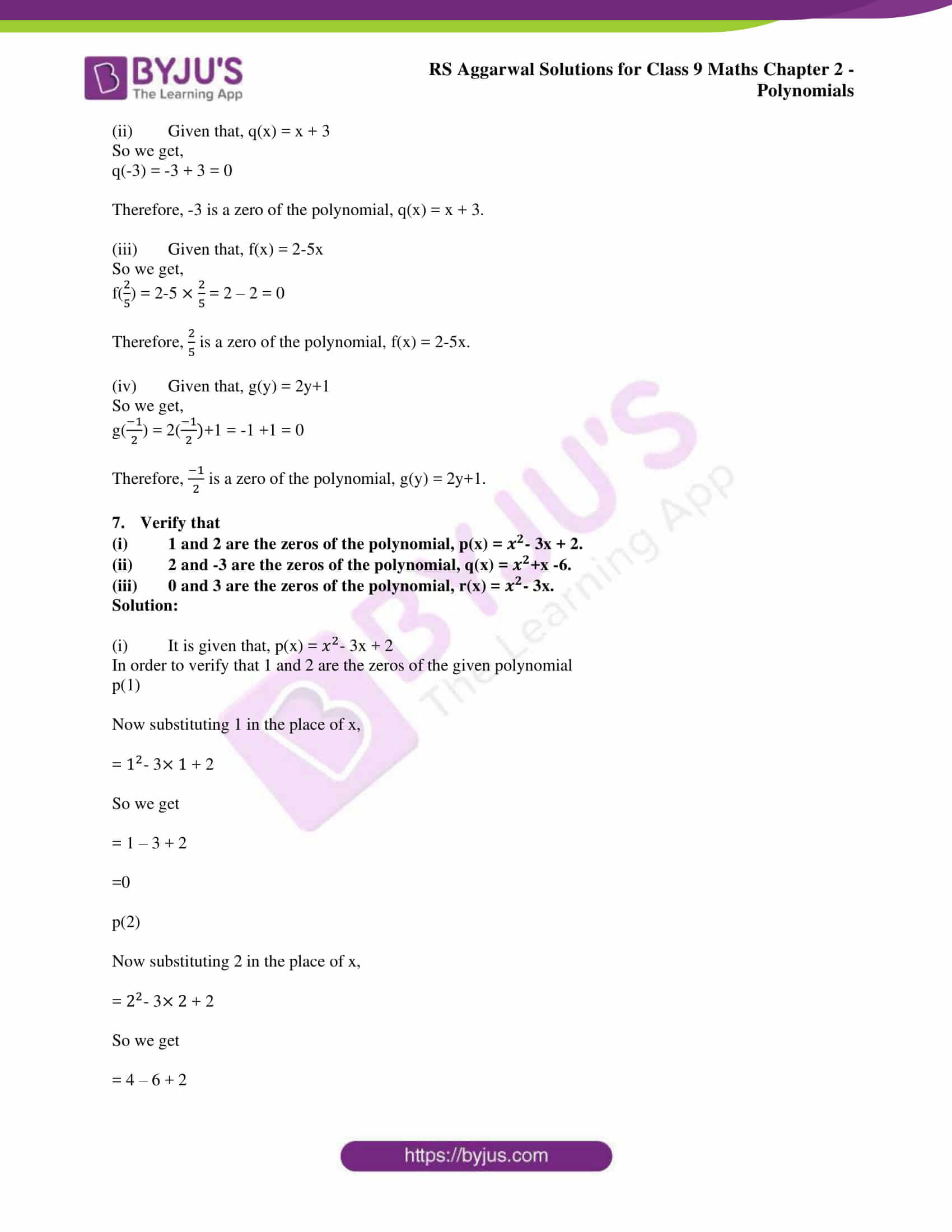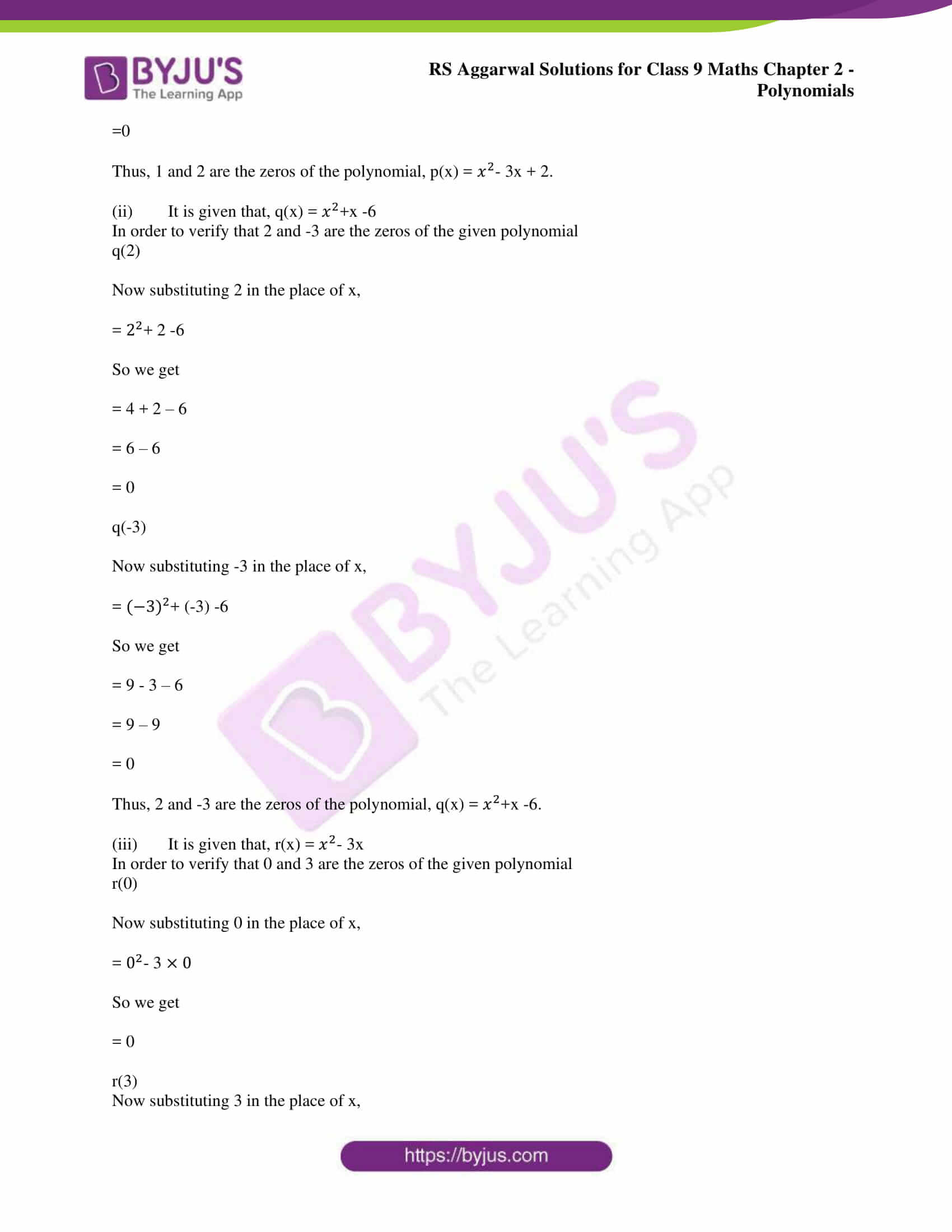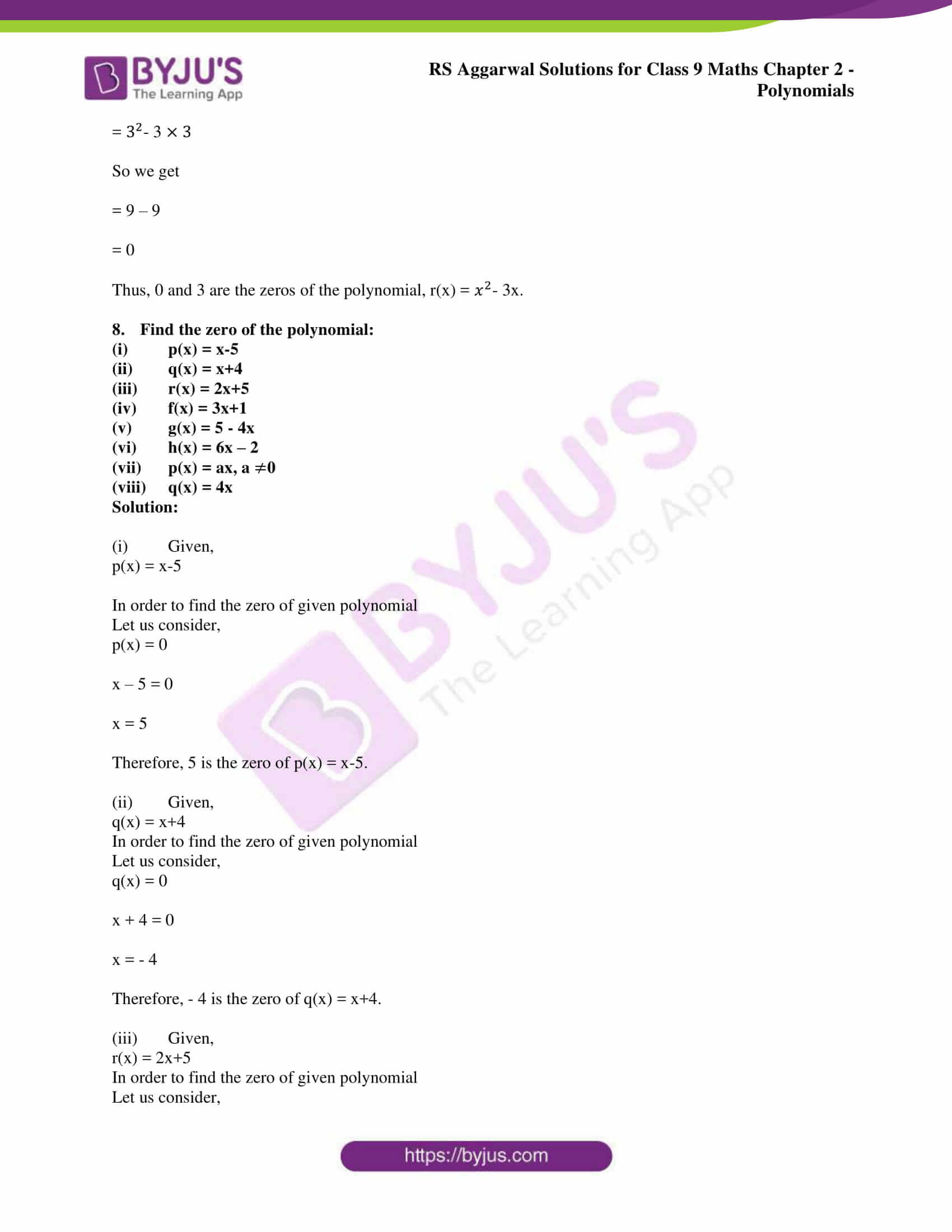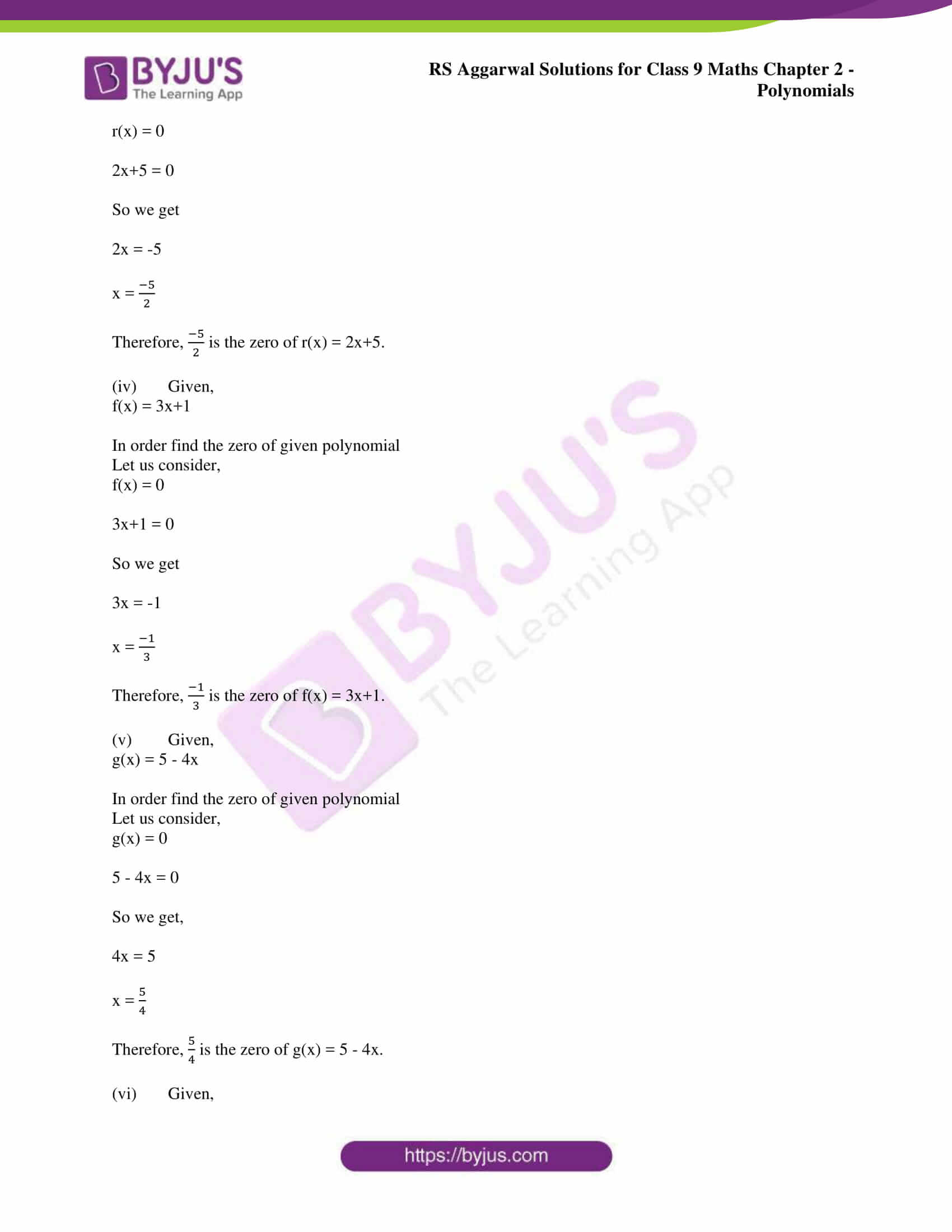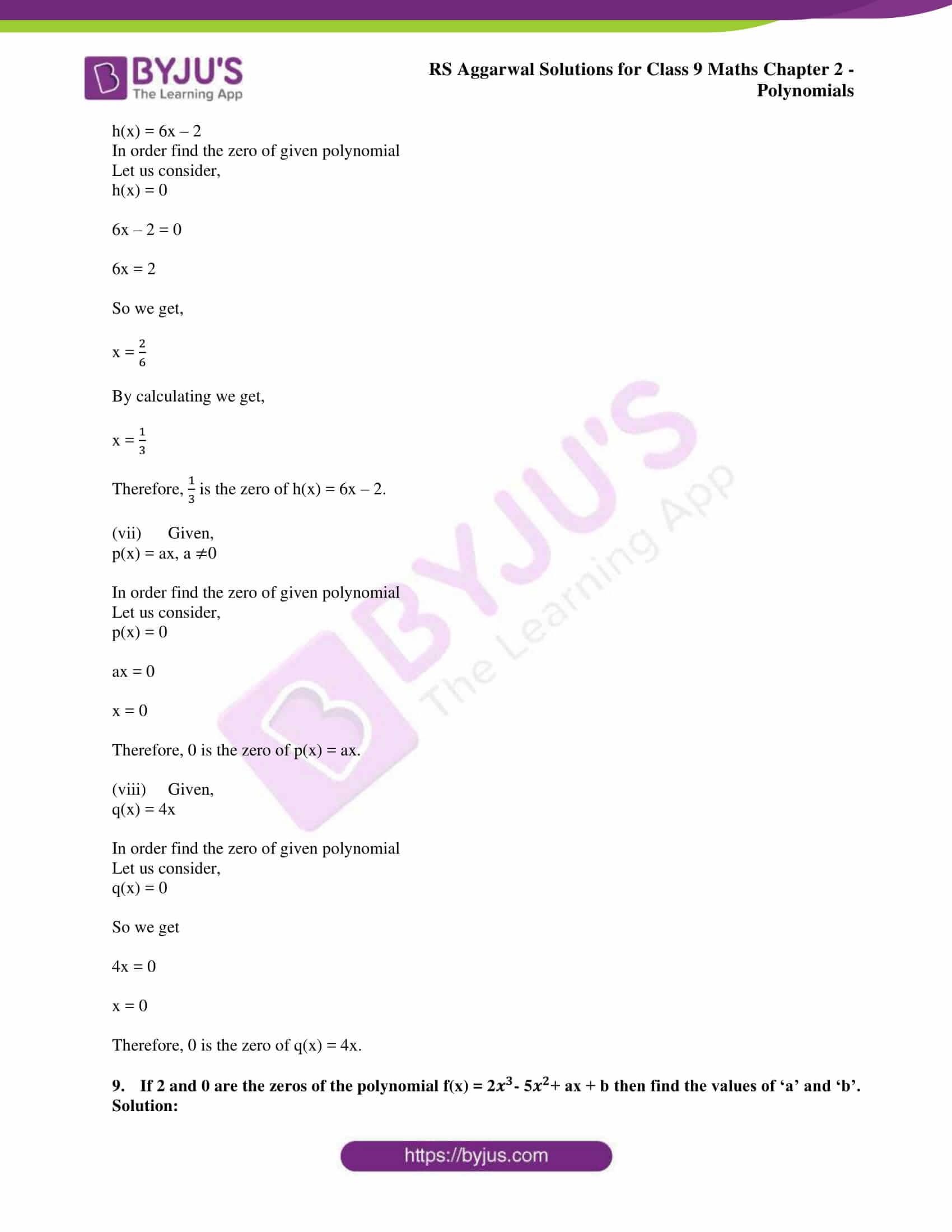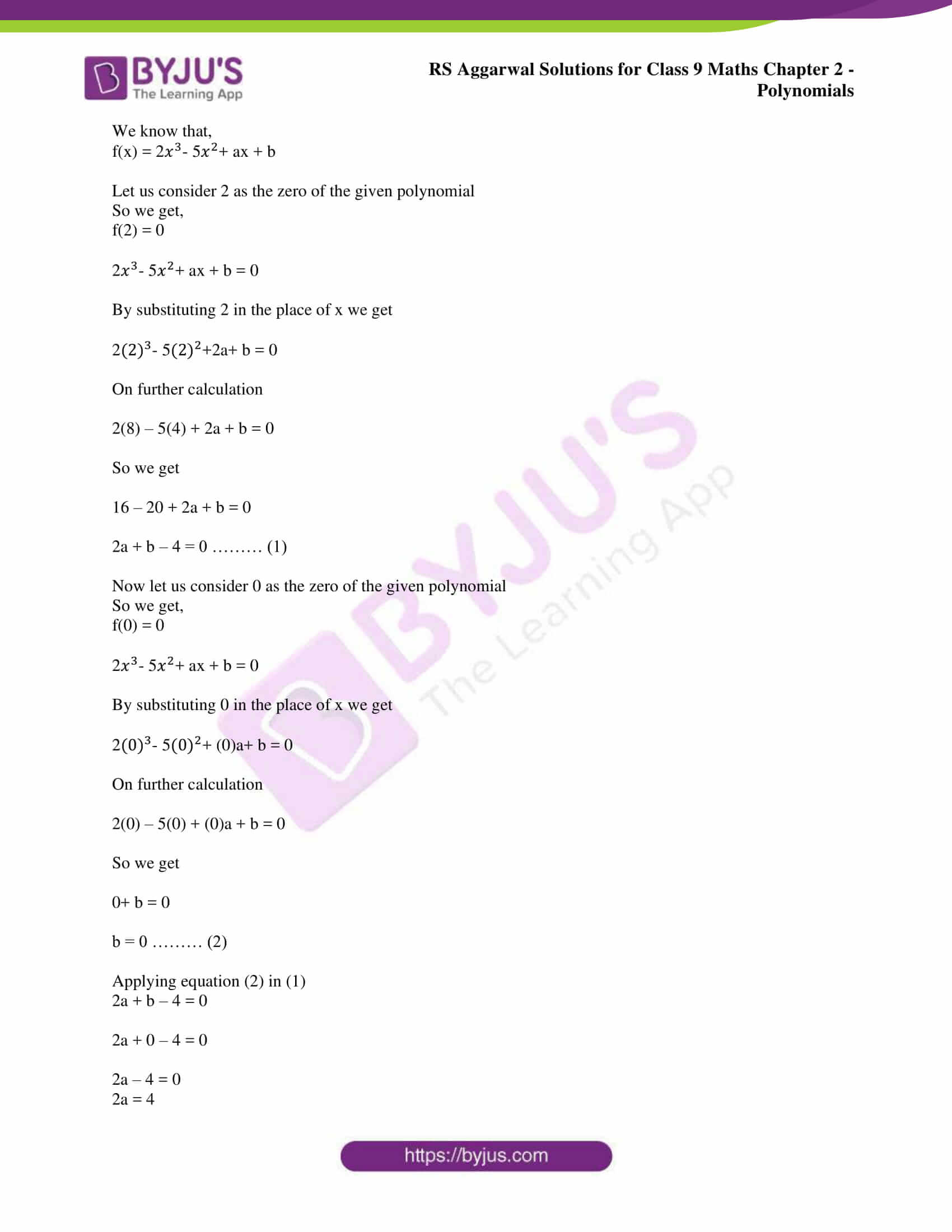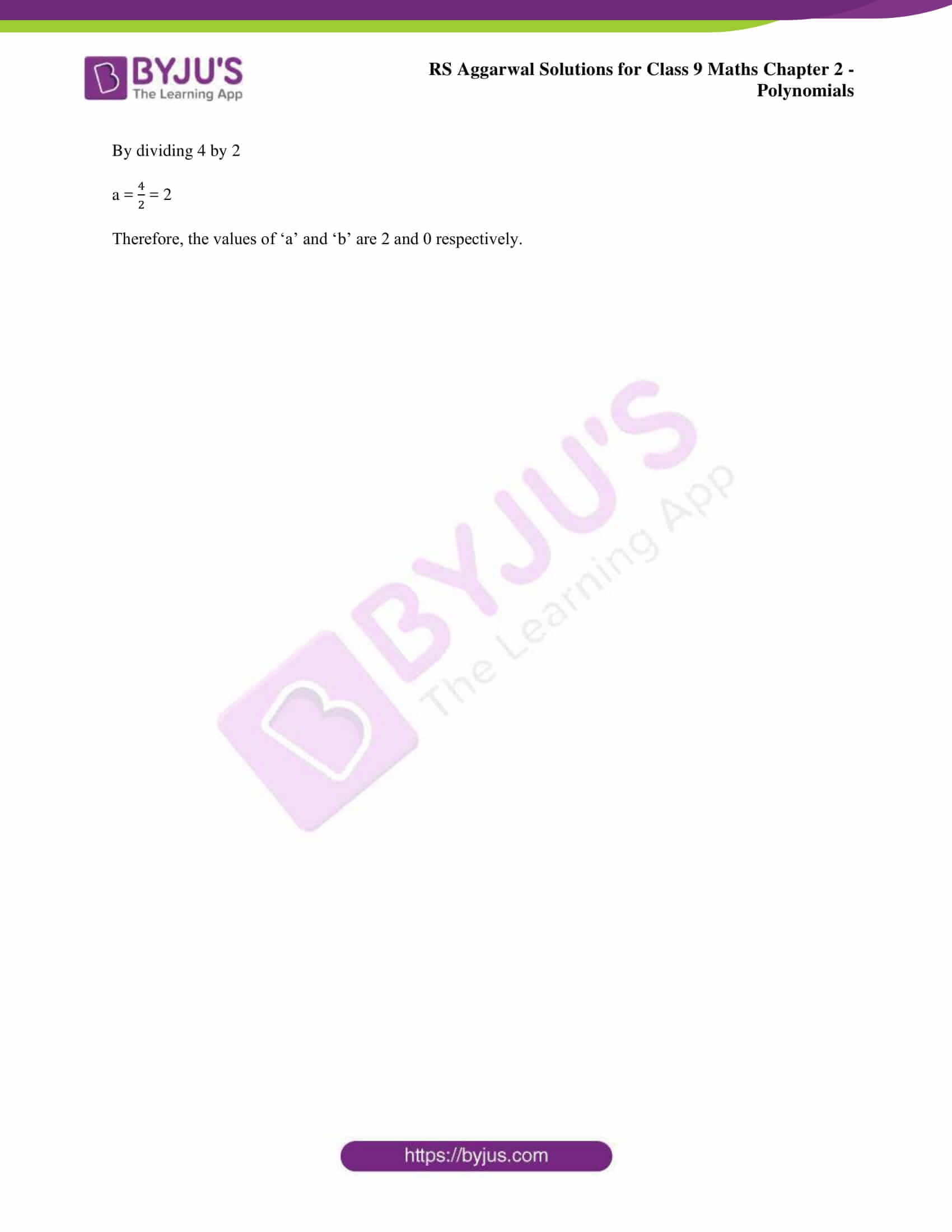### Access other exercise solutions of class 9 Maths Chapter 2: Polynomials

Exercise 2A Solutions 6 Questions

Exercise 2C Solutions 16 Questions

Exercise 2D Solutions 26 Questions

### RS Aggarwal Solutions Class 9 Maths Chapter 2 – Polynomials Exercise 2B

RS Aggarwal Solutions Class 9 Maths Chapter 2 Polynomials Exercise 2B contains ample number of examples and problems to find the value of a polynomial and zeros of a polynomial solved in simple methods according to the syllabus.

### Key features of RS Aggarwal Solutions for Class 9 Maths Chapter 2: Polynomials Exercise 2B

• The stepwise solutions of the problems in PDF format help students prepare for the exams faster.
• The students can analyse the time taken to solve each question and practise well to improve their speed.
• Every topic contains solved examples which makes it easier for the students while solving the exercises.
• Various methods used in solving the problems help students during revision to prepare for the exams.